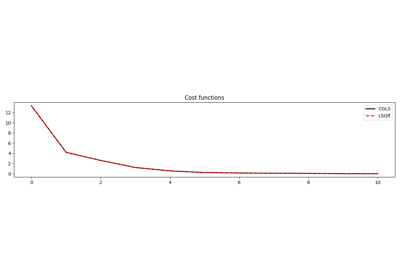# pylops.optimization.basic.lsqr#

pylops.optimization.basic.lsqr(Op, y, x0=None, damp=0.0, atol=1e-08, btol=1e-08, conlim=100000000.0, niter=10, calc_var=True, show=False, itershow=(10, 10, 10), callback=None)[source]#

LSQR

Solve an overdetermined system of equations given an operator Op and data y using LSQR iterations.

$\DeclareMathOperator{\cond}{cond}$
Parameters
Oppylops.LinearOperator

Operator to invert of size $$[N \times M]$$

ynp.ndarray

Data of size $$[N \times 1]$$

x0np.ndarray, optional

Initial guess of size $$[M \times 1]$$

dampfloat, optional

Damping coefficient

atol, btolfloat, optional

Stopping tolerances. If both are 1.0e-9, the final residual norm should be accurate to about 9 digits. (The solution will usually have fewer correct digits, depending on $$\cond(\mathbf{Op})$$ and the size of damp.)

conlimfloat, optional

Stopping tolerance on $$\cond(\mathbf{Op})$$ exceeds conlim. For square, conlim could be as large as 1.0e+12. For least-squares problems, conlim should be less than 1.0e+8. Maximum precision can be obtained by setting atol = btol = conlim = 0, but the number of iterations may then be excessive.

niterint, optional

Number of iterations

calc_varbool, optional

Estimate diagonals of $$(\mathbf{Op}^H\mathbf{Op} + \epsilon^2\mathbf{I})^{-1}$$.

showbool, optional

Display iterations log

itershowtuple, optional

Display set log for the first N1 steps, last N2 steps, and every N3 steps in between where N1, N2, N3 are the three element of the list.

callbackcallable, optional

Function with signature (callback(x)) to call after each iteration where x is the current model vector

Returns
xnp.ndarray

Estimated model of size $$[M \times 1]$$

istopint

Gives the reason for termination

0 means the exact solution is $$\mathbf{x}=0$$

1 means $$\mathbf{x}$$ is an approximate solution to $$\mathbf{y} = \mathbf{Op}\,\mathbf{x}$$

2 means $$\mathbf{x}$$ approximately solves the least-squares problem

3 means the estimate of $$\cond(\overline{\mathbf{Op}})$$ has exceeded conlim

4 means $$\mathbf{y} - \mathbf{Op}\,\mathbf{x}$$ is small enough for this machine

5 means the least-squares solution is good enough for this machine

6 means $$\cond(\overline{\mathbf{Op}})$$ seems to be too large for this machine

7 means the iteration limit has been reached

r1normfloat

$$||\mathbf{r}||_2^2$$, where $$\mathbf{r} = \mathbf{y} - \mathbf{Op}\,\mathbf{x}$$

r2normfloat

$$\sqrt{\mathbf{r}^T\mathbf{r} + \epsilon^2 \mathbf{x}^T\mathbf{x}}$$. Equal to r1norm if $$\epsilon=0$$

anormfloat

Estimate of Frobenius norm of $$\overline{\mathbf{Op}} = [\mathbf{Op} \; \epsilon \mathbf{I}]$$

acondfloat

Estimate of $$\cond(\overline{\mathbf{Op}})$$

arnormfloat

Estimate of norm of $$\cond(\mathbf{Op}^H\mathbf{r}- \epsilon^2\mathbf{x})$$

varfloat

Diagonals of $$(\mathbf{Op}^H\mathbf{Op})^{-1}$$ (if damp=0) or more generally $$(\mathbf{Op}^H\mathbf{Op} + \epsilon^2\mathbf{I})^{-1}$$.

costnumpy.ndarray, optional

History of r1norm through iterations

Notes

## Examples using pylops.optimization.basic.lsqr#CGLS and LSQR Solvers

CGLS and LSQR Solvers